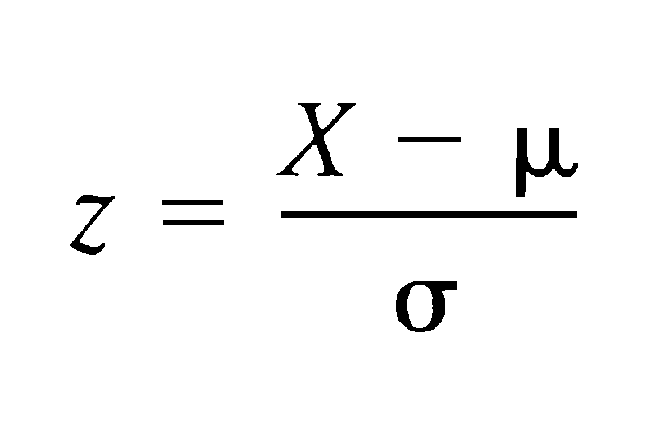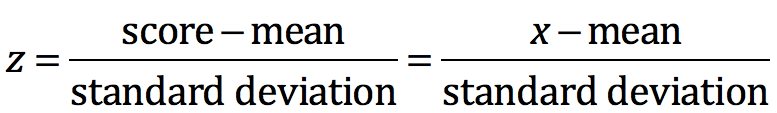Search

# Interpret the z-score (Like it’s Your Job)Most of our students will have no trouble calculating a z-score. But interpreting the z-score is a much more important concept for their future success. Here is one approach to get them there:

Rather than shoving the formula in front of students, try to put them in a situation where they discover the need for the concept of a z-score. Students are given this scenario and asked to discuss in pairs:

The Chapter 1 AP Statistics test had an average of 70 and a standard deviation of 10 while the Chapter 1 AP Psychology test had an average of 70 and a standard deviation of 5. Luke scored a 90 on the AP Statistics test and an 85 on the AP Psychology test. Which of Luke’s test scores is more impressive?

AP Stats test.      Mean = 70     SD = 10          Luke test score = 90

AP Psych test.     Mean = 70     SD = 5            Luke test score = 85

Of course at first glance, the AP Stats test looks more impressive as 90 > 85. But some students will start to realize that the AP Psych test is actually more impressive, as the score is 3 full standard deviations above the mean while the AP Stats score is only 2 standard deviations above the mean.

When students share out their thoughts on this short activity, ask them to explain the (simple) mathematics they used to arrive at 3 and 2, and we get this:At this point, don’t worry at all about using Greek letter notation, as we are building a concept.

## Develop the concept of the z-score

Next, we calculate the z-score for several students for the AP Stats test (mean = 70, SD = 10).

Luke test score: 90                z-score = 2

Biff test score: 60                  z-score = -1

Marty test score: 88              z-score = 1.8

In pairs, have students discuss the meaning of the z-score. What is a z-score of 1.8 telling us? And we arrive at the goal of the lesson:

Student: “Marty’s Chapter 1 test score is 1.8 standard deviations above the mean”

## Make it someone’s job

Because the z-score interpretation is so important, I hire a student to be in charge of this interpretation. This is a full year commitment and is a paid position (\$1 paid in full at the end of the year…but it is a great resume builder).